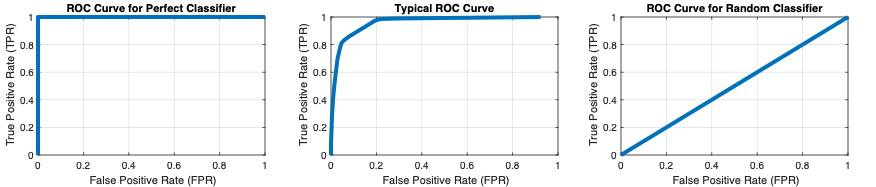# ROC Curve

## Evaluate the performance of machine learning classification models

ROC curves (receiver operating characteristic curves) are an important tool for evaluating the performance of a machine learning model. They are most commonly used for binary classification problems – those that have two distinct output classes. The ROC curve shows the relationship between the true positive rate (TPR) for the model and the false positive rate (FPR). The TPR is the rate at which the classifier predicts “positive” for observations that are “positive.” The FPR is the rate at which the classifier predicts “positive” for observations that are actually “negative.” A perfect classifier will have a TPR of 1 and an FPR of 0.

You can calculate ROC curves in MATLAB® using the `perfcurve` function from Statistics and Machine Learning Toolbox™. Additionally, the Classification Learner app generates ROC curves to help you assess model performance. The app lets you specify different classes to plot, so you can view ROC curves for multiclass classification problems that have more than two distinct output classes.

Video length is 4:43.

### How ROC Curves Work

Most machine learning models for binary classification do not output just 1 or 0 when they make a prediction. Instead, they output a continuous value somewhere in the range [0,1]. Values at or above a certain threshold (for example 0.5) are then classified as 1 and values below that threshold are classified as 0. The points on the ROC curve represent the FPR and TPR for different threshold values.

The selected threshold can be anywhere on the range [0,1], and the resulting classifications will change based on the value of this threshold. For example, if the threshold is set all the way to 0, the model will always predict 1 (anything at or above 0 is classified as 1) resulting in a TPR of 1 and an FPR of 1. At the other end of the ROC curve, if the threshold is set to 1, the model will always predict 0 (anything below 1 is classified as 0) resulting in a TPR of 0 and an FPR of 0.

When evaluating the performance of a classification model, you are most interested in what happens in between these extreme cases. In general, the more “up and to the left” the ROC curve is, the better the classifier.

ROC curves are typically used with cross-validation to assess the performance of the model on validation or test data .ROC curves calculated with the `perfcurve` function for (from left to right) a perfect classifier, a typical classifier, and a classifier that does no better than a random guess.